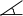# Level 3 - Math & Problem Solving

Our Level 3 Math & Problem Solving program typically targets students in Grades 7 through 9. However, younger students may, albeit rarely, be placed at Level 3 if they meet all prerequisites for this level. This level addresses, in depth, all four steps of problem solving by applying these to quite complex and difficult problems.

Level 3 instruction includes, but is not limited to:

###### Math
• Numerical systems: Egyptian, Roman, base 2, base 5, base 8, base 12, and other
• Elements of formal logic (Boolean Algebra)
• Equations and systems of equations
• Difficult problems on percent and proportions
• Electrical circuits for simple Boolean operators
• Algebra: complex problems on one variable
and on two variables
• Elements of geometry
• Tricky problems on exponents
###### Logic and Problem-Solving Strategies
• Modeling problems and simplifying
• Forward solving strategies
• Backward solving strategies
• Venn diagrams
• Logical charts
• Organized / Systematic list
• Problems on lowest common multiple
• Logical tree
• Problem decomposition
• Probability and possibility

And much more!!!  Games, puzzles, history of mathematical theorems, and hands-on fun!

Prerequisite for Level 3: Knowledge of positive and negative numbers, elementary algebra, and problem-solving strategies and techniques from Level 2; OR successful completion of interview (for new students).

##### Sample Problems

Last week, a store owner received a supply of cans of pickled cucumbers in 7 large boxes and 3 small boxes. An invoice indicated that there were 108 cans altogether in these boxes. This week the store owner received 5 large boxes and 4 small boxes of pickled cucumbers. This week the invoice states that there are 92 cans of pickled cucumbers altogether. The store owner does not want to open these boxes but he is keen to know how many cans are in each large box. Despite his lack of knowledge in algebra, the store owner managed to figure this out.

• Can you figure out how many cans are in each large box?

Add/Subtract in Egyptian numerals:Given external angle EAB. AT is a tangent line; length of AT = 18 cm.P = 28°;S = 74°. Length of AB = 6 cm, and length of ED = 77 cm.

• Find out the value ofEAB, and the length of EA and CA.